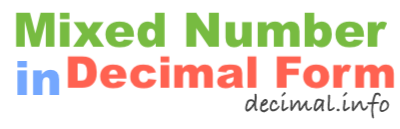What is 3 1/8 in decimal form?To start off, note that 3 1/8 is a mixed number, also known as a mixed fraction. It has a whole number and a fractional number. We have labeled the parts of the mixed number below so it is easier to follow along.

3 = Whole number
1 = Numerator
8 = Denominator

To get 3 1/8 in decimal form, we basically convert the mixed number to a fraction and then we divide the numerator of the fraction by the denominator of the fraction.

Here are the detailed math steps we use to convert 3 1/8 mixed number to decimal form:

Step 1: Multiply the whole number by the denominator:

3 × 8 = 24

Step 2: Add the product you got in Step 1 to the numerator:

24 + 1 = 25

Step 3: Divide the sum from Step 2 by the denominator:

25 ÷ 8 = 3.125

That's it folks! The answer to 3 1/8 in decimal form is displayed below:

3 1/8 ≈ 3.13

Mixed Number in Decimal Form
3 1/8 in decimal form is not all we can do! Here you can convert another mixed number to decimal form.

What is 3 1/9 in decimal form?
Here is the next mixed number on our list that we have converted into decimal form.